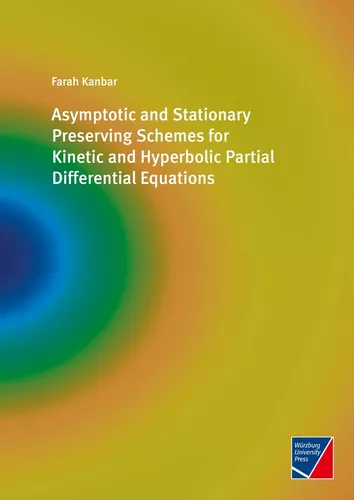# Asymptotic and Stationary Preserving Schemes for Kinetic and Hyperbolic Partial Differential Equations

Natur- & Humanwissenschaften

Paperback

152 Seiten

ISBN-13: 9783958262102

Verlag: Würzburg University Press

Erscheinungsdatum: 09.05.2023

Sprache: Englisch

Farbe: Ja

Bewertung::
0%
29,80 €

inkl. MwSt. / portofrei

Ihr eigenes Buch!

Werden Sie Autor*in mit BoD und erfüllen Sie sich den Traum vom eigenen Buch und E-Book.

Mehr erfahren
In this thesis, we are interested in numerically preserving stationary solutions of balance laws. We start by developing finite volume well-balanced schemes for the system of Euler equations and the system of Magnetohydrodynamics (MHD) equations with gravitational source term. Since fluid models and kinetic models are related, this leads us to investigate Asymptotic Preserving (AP) schemes for kinetic equations and their ability to preserve stationary solutions.
In an attempt to mimic our result for kinetic equations in the context of fluid models, for the isentropic Euler equations we developed an AP scheme in the limit of the Mach number going to zero. The properties of the schemes we developed and its criteria are validated numerically by various test cases from the literature.Farah Kanbar

geboren in Kfarremman, Libanon, M.Sc. (Mathematik)

Es sind momentan noch keine Pressestimmen vorhanden.

Eigene Bewertung schreiben
Bitte melden Sie sich hier an, um eine Rezension abzugeben.
Suchmaschine unterstützt von ElasticSuite